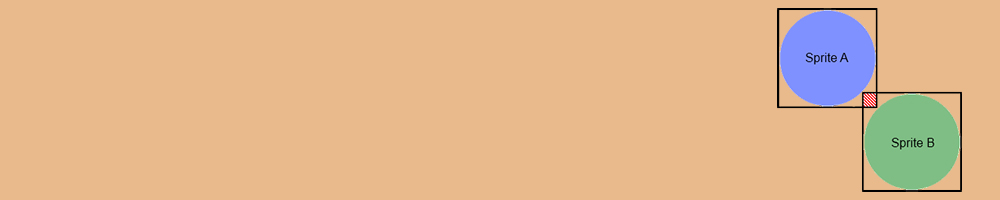# Collision Detection using Custom Bounding Rectangle/BoxPermalink

While working on the client project I came cross to implement a module using kineticjs framework. In this module the users can draw multiple points polygon shapes. In each drawings and changes on the polygon the shapes need to be sorted according to the ascending order of the shape areas. Since it deals with many polygon shapes it required to implement an algorithm to faster the ordering or position of the Z index in 2D perspective view.

To solve this problem:

• After each update of polygon shapes retrieve all the shapes form the drawing canvas.
• Then calculate the min and max vertex points of each polygon. So basically it’s like you will get to point where we can draw virtual rectangle or box shape.
• After calculating the min and max vales of each polygon check for the shape collision. In simple term check for each min and max points of shape is intersecting with other shape min and max points. Here you can find a simple code for virtual collision detection for multiple point shapes.

Once you have detected the collision/Shapes are overlapping each other then only need is to calculate areas of each colliding shapes. This will help to reduce the computational time and faster the process.

The below code is to calculate the area of multiple point shape polygon.

Now that’s all. According the areas size sort the Z index position in ascending order.

Note that since it is dealing with multiple shapes it is must this logic need to be run as a recursive function so until it get sorted and match with all the shapes.

Happy Coding :)